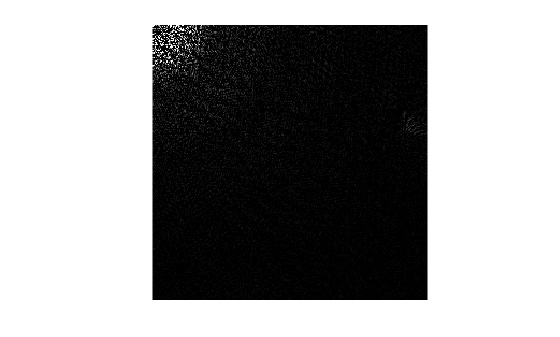# dctmtx

Discrete cosine transform matrix

## Syntax

``D = dctmtx(n)``

## Description

example

````D = dctmtx(n)` returns the `n`-by-`n` discrete cosine transform (DCT) matrix, which you can use to perform a 2-D DCT on an image.```

## Examples

collapse all

Read an image into the workspace and cast it to class `double`.

```A = im2double(imread('rice.png')); imshow(A)```Calculate the discrete cosine transform matrix.

`D = dctmtx(size(A,1));`

Multiply the input image `A` by `D` to get the DCT of the columns of `A`, and by `D'` to get the inverse DCT of the columns of `A`.

```dct = D*A*D'; imshow(dct)```## Input Arguments

collapse all

Size of DCT matrix, specified as a positive integer.

Data Types: `double`

## Output Arguments

collapse all

DCT matrix, returned as a numeric matrix of size `n`-by-`n`.

Data Types: `double`

## Tips

• If you have an `n`-by-`n` image, `A`, then `D*A` is the DCT of the columns of `A` and `D'*A` is the inverse DCT of the columns of `A`.

• The two-dimensional DCT of `A` can be computed as `D*A*D'`. This computation is sometimes faster than using `dct2`, especially if you are computing a large number of small DCTs, because `D` needs to be determined only once.

For example, in JPEG compression, the DCT of each 8-by-8 block is computed. To perform this computation, use `dctmtx` to determine `D`, and then calculate each DCT using `D*A*D'` (where `A` is each 8-by-8 block). This is faster than calling `dct2` for each individual block.